A tutorial on Register Allocation by Puzzle Solving. This page contains a gentle introduction on register allocation by puzzle solving, in the form of a slide show. Puzzles are a new way of looking at the old register allocation problem. The main reference on this topic is the PLDI'08 paper, as well as his longer sibling. You can click on any of the images to see its bigger version.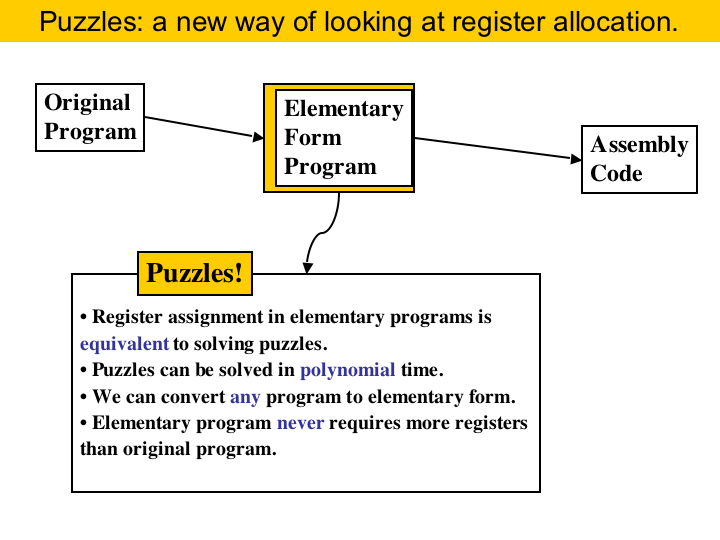Puzzles are a new abstraction for doing register allocation. This page describes the program representation called elementary form, and shows that doing register allocation in elementary programs is equivalent to solving some types of puzzles. There are many advantages in this approach: puzzles can be solved in polynomial time, we can convert any program into elementary form, and elementary programs never require more registers than the original program.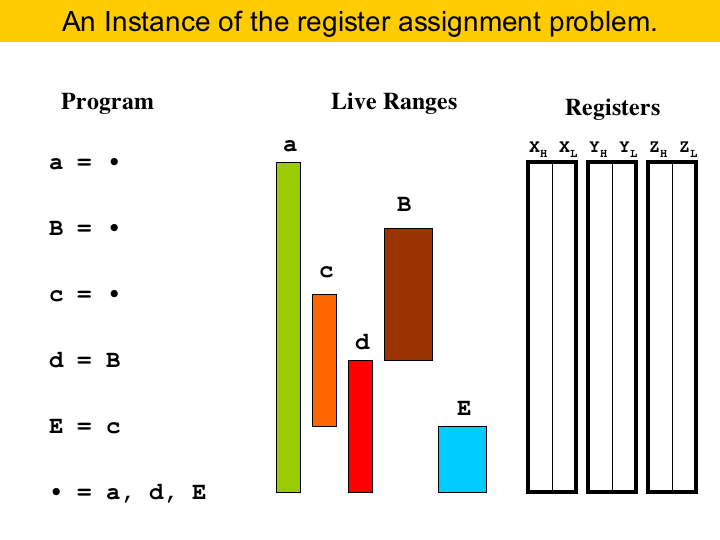When we compile a program, we must find locations to store the values used in that program. The compiler has basically two options: either it places the values in memory, or it puts them into registers. Registers in general are better, because they tend to be much faster than memory. The problem is that they come in small number... Thus, register allocation is basically this problem of trying to map registers to variables. The constraint, in this case, is the live ranges of the variables. The live range of a variable is the set of program points where that variable is live, that is, it is the set of program points between the instruction that defines the variable, until all the instructions that use that variable. For instance, here we have a program, without branches, so it is a straight line piece of code, and the live ranges of the variables are the colored bars. In the right of the figure we have the registers. Let's assume that we are targeting an architecture with register pairs. This means that two small registers combine to form a big register. For example, register X is formed by register XH, its higher half, and register XL, its lower half. We may notice that some variables are thick, like B and E. They must be stored into a big register. The other variables are thin, and they can be stores into a half.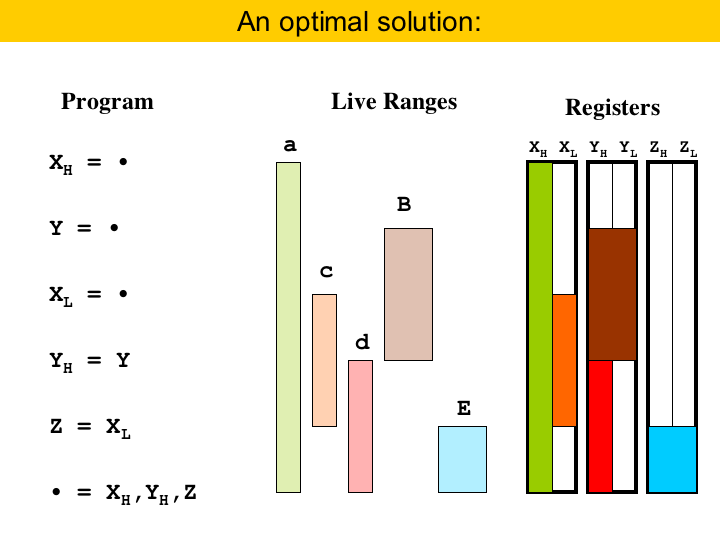And here we have a solution for this allocation problem. The empty bars represent the state of the registers at different times during the program execution. This solution is optimal, in the sense that we cannot use less than three registers to solve this program. By the way, this problem is NP-complete (Lee'07). That is, if we have a bunch of lines like this, the problem of packing them into registers is NP-complete. If the lines had all the same width, then we could do this in polynomial time. But there is a sad thing going on here. Towards the end of the program, variables a and d, green and red, end up occupying different registers, even though they would fit into the same register. Because of this, the thick variable E had to be placed into a third register. Our abstraction allows to solve this problem!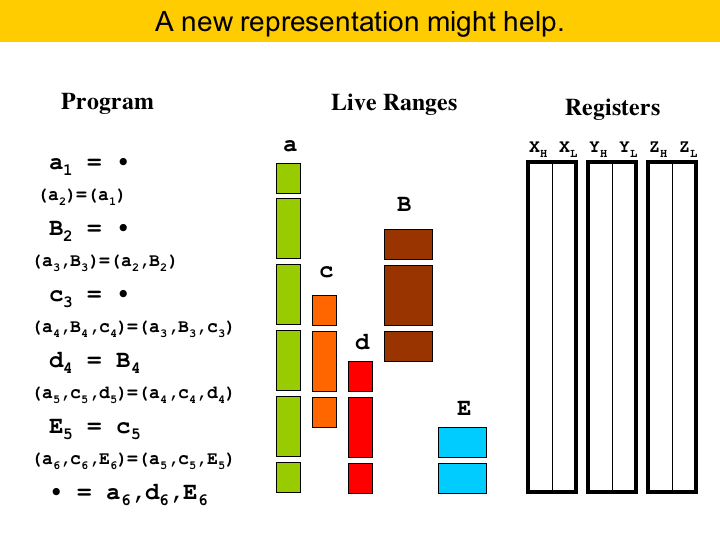So, what do we propose? Well, to facilitate solving the register allocation problem, we propose to do some very aggressive form of live range splitting. The idea is that, if live ranges are shorter, it is easier to fit them into registers. So, to do register allocation, we will adopt a different program representation. We call this representation Elementary-Form. It is not new: Appel and George used elementary-form in their register allocator based on integer linear programming (Appel'01). The good thing about this representation is that live ranges are very, very small. If you see in the figure, each live range contains at most two program points, where a program point is the point between two consecutive instructions.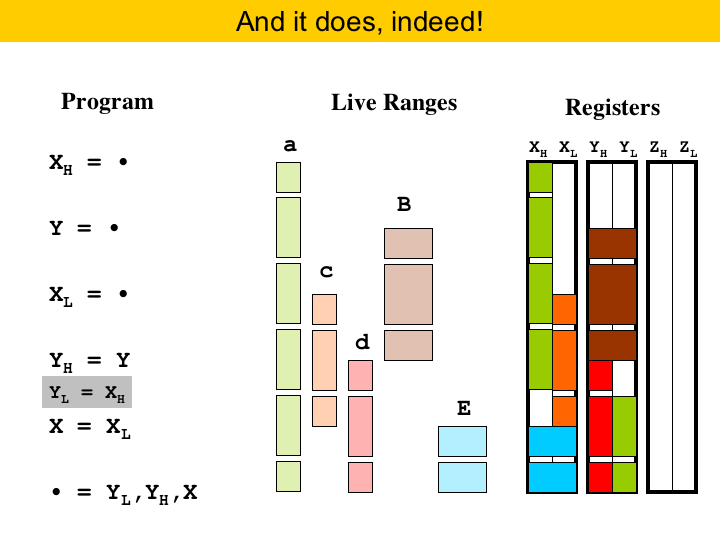Here we have the result of the register allocation. We are using only two registers, whereas before we needed three. One curious thing is the allocation of 'a'. It starts assigned to register XH, but then, before the second to last instruction, 'a' is moved to another register, in this case YL. This allocation is optimal. We can prove that, if the live ranges are always so small, we can always find the optimal allocation in polynomial time. Of course, there is no free lunch, and to move variables around registers, sometimes we have to insert some instructions in the target code. This copy, marked in gray here, was inserted to move variable 'a'.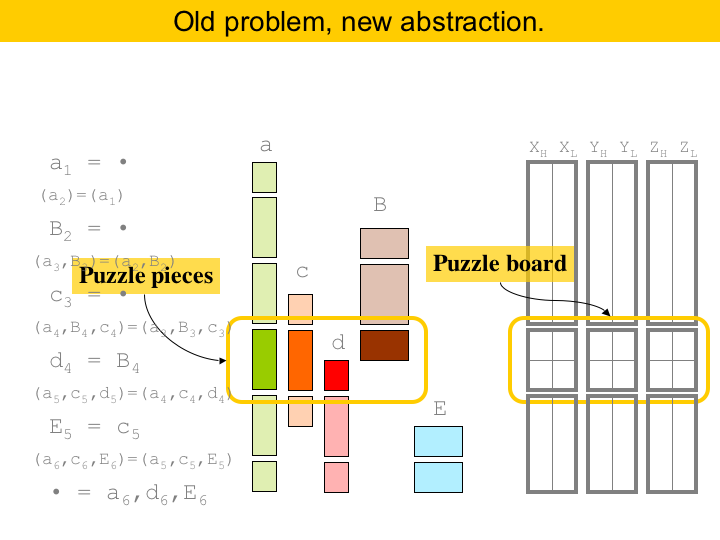In essence, what we are proposing is a new abstraction to deal with register allocation. We call our abstraction puzzles. Roughly, we will be producing one puzzle per program instruction. We have highlighted one puzzle in the figure. The puzzle board is given by the register bank. In this example, the board has three areas, each one divided into two columns and two rows. The pieces of our puzzle are given by the program variables. For example, the highlighted puzzle has four pieces. We have some rules about where to put pieces. The brown piece, representing variable 'B', can be placed only on the upper row of the area. We are free to choose which area though. The little red piece, representing variable 'd', can only be inserted somewhere in the lower row. The green and orange pieces span both rows. They occupy only one column though. The good thing is that we can solve puzzles optimally in polynomial time, even if some areas of the puzzle are initially taken. Also, any program can be placed into elementary form, and, on the good side, the elementary program will never require more registers than the original program!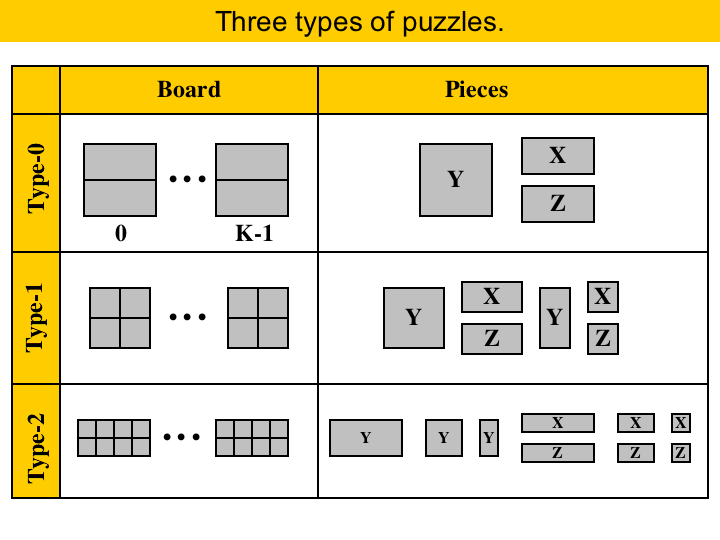A puzzle consists of a board and a set of pieces. The challenge is to fit all the pieces on the board. Pieces cannot overlap, and some pieces are already placed on the board. Every puzzle board has a number of separate areas, where each area is divided into two rows of squares. We have identified three types of puzzles that are important for register allocation. We call them Type-0, Type-1 and Type-2. The type of a puzzle is determined by its board, and the shape of its pieces. Type-0 puzzles are very simple: each area has only one column. It contains only three types of pieces, that we will call Y, X and Z. We can place a X-piece on any square in the upper row, we can place a Z-piece on any square in the lower row, and we can place a Y-piece on any column. The algorithm to solve type-0 puzzles is very trivial, so we leave it for the reader as an assignment. Type-1 puzzles have areas with two columns, and six different kinds of pieces. The rules here are similar to type-0: X pieces go into the upper row, Z pieces go into the lower row. However, we have some thicker pieces now. A piece that spans two columns must be completely inside an area. This means that we cannot put half a piece in an area, and half in another area. We will describe a linear-time algorithm to solve type-1 puzzles later. Type-2 puzzles have areas with four columns, and nine types of pieces. The only thing special here is that pieces of width two must be placed either on the first half of an area, or on the second half. Solving type-2 puzzles is still an open-problem.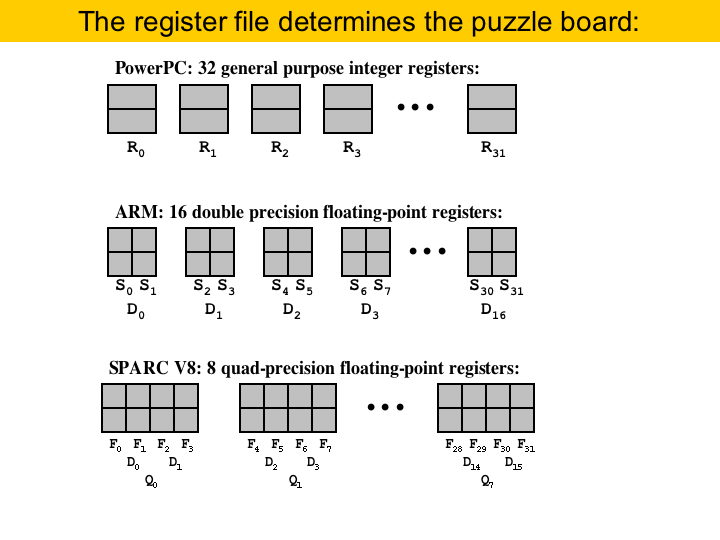The question that someone may be asking now is what determines the type of a register allocation puzzle; and the answer is: the bank of registers. The possibility of grouping registers into pairs, or quadruples gives the number of columns in the puzzle board. Two registers alias if assigning a value to one can change the other. In PowerPC we have no aliasing: each register has just one name, so, the register bank of PowerPC produces a type-0 puzzle. The same is true about the integer registers in ARM. On the other hand, consider the floating point registers of ARM. We have either single precision registers or double precision registers. Two single precision combine to make a double precision, and then we have the two columns in each area of a type-1 puzzle. Type-2 puzzles are more rare. An example is found in the floating point bank of Ultra-Sparc. In Sparc v8 we have single, double and quadruple precision floating point registers. So, the areas of our puzzle would have four columns. One point that must be made clear is that each column in an area has a name in the register bank of the target architecture. So, because in PowerPC we have registers R0 to R31, that is how the columns in a PowerPC puzzle are called. In the same way, because in ARM the floating point registers S0 and S1 combine to form the double D0, that is the name of the columns of the first area of a ARM puzzle.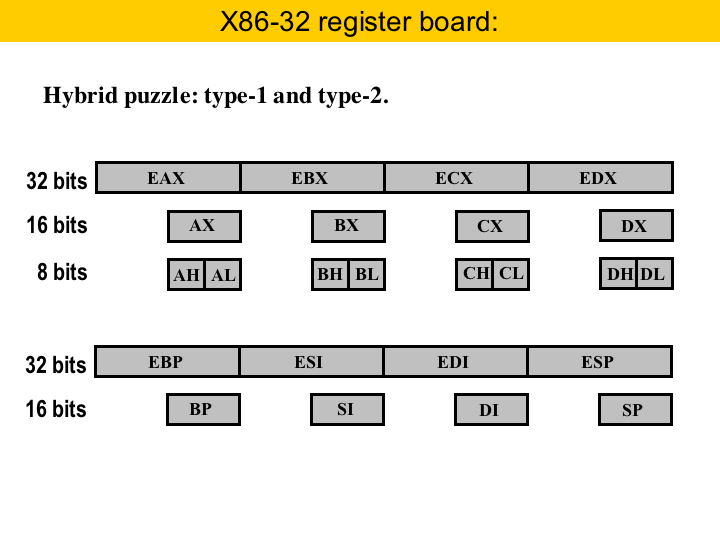We can have also hybrid puzzles, and the canonical example is the general purpose register bank of the 32-bit X86 machine. This is a hybrid of type-0 and type-1. The registers AX, BX, CX and DX give the type-1 part. Each of these registers is 16-bits wide, and alias two 8-bit registers. For instance, AX alias AL, its lower part, and AH, its higher part. What about the extended registers: EAX, EBX, etc? Those are 32-bit registers. So, AX and EAX are aliased, for instance. Well, for all the purposes, we can treat AX and EAX as the same register, when solving puzzles, because they encompass the same pairs: AL and AH. The registers BP, SI, DI and SP give the type-0 part of the puzzle board. These registers do not contain pairs. To solve this type of puzzle, we can solve each part in separate. That is, first we solve the type-0 part, and after that, we solve the type-1 part.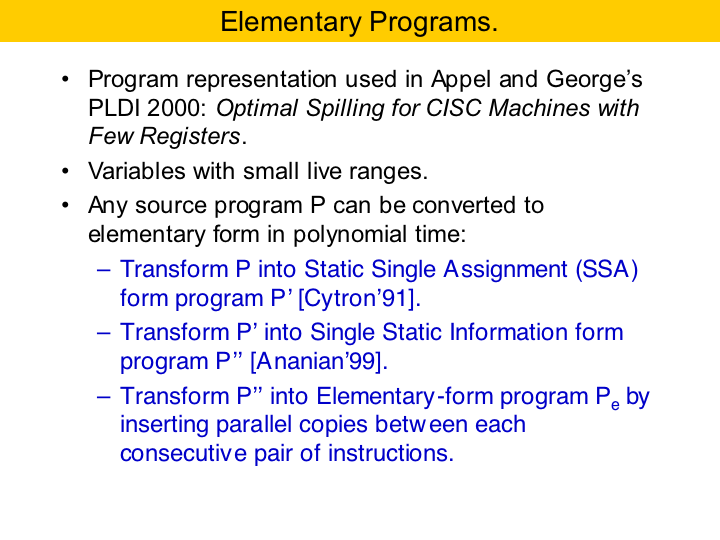We are getting closer of talking about pieces and rows, but before, let's define elementary-form, for that is where the pieces and rows come from. Elementary-form is a program representation that is a subset of Static Single Assignment Form (SSA-form to be short). This representation had been used before. Not with this name, but the idea was the same. Maybe some of you will remember a paper that Andrew Appel and Lal George had in PLDI 2000: the title was Optimal Spilling for CISC Machines with Few Registers. In that paper they divided the program between each pair of consecutive instructions. The idea was to allow a variable to be alive at some program points and not at others. Any program can be converted into elementary form in three steps. First we convert it to SSA-form. Second, we convert the program to SSI-form (Ananian'99). This is Static Single Information form. SSI-form was the master thesis of Scott Ananian, from MIT. The idea was to split the live range of variables when they leave a basic block. SSI is a subset of SSA-form. Then, after putting the program into SSI-form we get elementary form by inserting parallel copies between each pair of consecutive instructions, and then doing variable renaming.Here we have a complete example. In the left we have the original program. This is a simple program, with four basic blocks. Each program point is indicated in red. In the right we have the same program in elementary form. So, how do we get from the program in the left to the program in the right? First, to convert into SSA-form we had to rename variables that are defined more than once, and we have to insert phi-functions to joy live ranges that converge into the same basic block. We inserted one phi-functio here, in green. The next step is to convert the program to SSI-form. So, every time the live range of a variable branches into two basic blocks, we have to redefine the variable at the beginning of each basic block. We do this redefinition with pi-functions. They are the duals of phi-functions. That is, whereas the phi-function join the live ranges that represent the same variable, the pi-functions split the live ranges that represent the same variables. The pi-functions, in this example, are colored in blue. Finally, we add the parallel copies between pairs of consecutive instructions. Here they are painted in red. We rename the variables in and out of parallel copies. After this step, each live range contains at most two program points.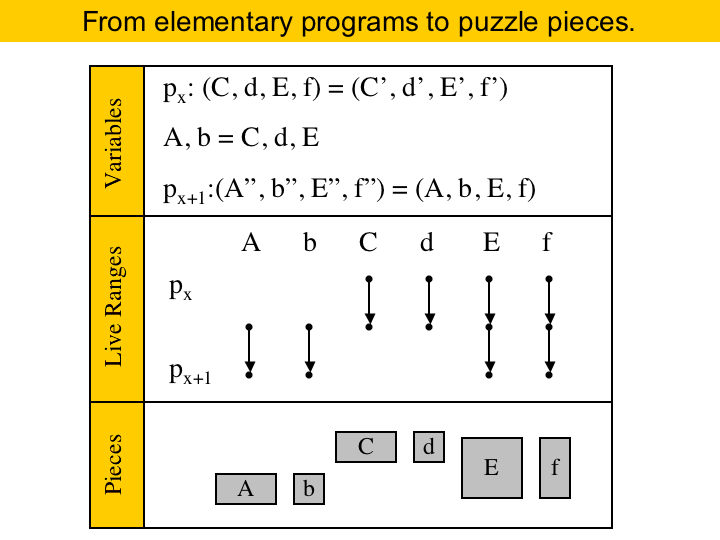We call the point between two consecutive instructions a program point. The live range of a variable is the collection of program points where that variable is alive. In elementary form, each live range includes at most two program points. We will say that a variable is live-in at an instruction if its live range contains the program point that precedes that instruction. Live-out if the live range contains the program point after the instruction. For each instruction we will create a puzzle. The board, as said before, is given by the register bank. The pieces are given by the live ranges of the variables alive across that instruction. If a live range of a variable ends at the instruction, it becomes a X piece. If it starts at the instruction, then it becomes a Z piece. If it goes through the instruction, then it becomes a Y piece. The width of a piece depends on the size of the register necessary to store that variable. For instance, in ARM the thick pieces would be doubles, and the thin pieces would be floats. Here we have an example. A, C and E are double-precision, and the other pieces are single-precision. This instruction defines two variables, A and b, and these variables produce Z pieces. The instruction also kills variables C and d, which become X pieces.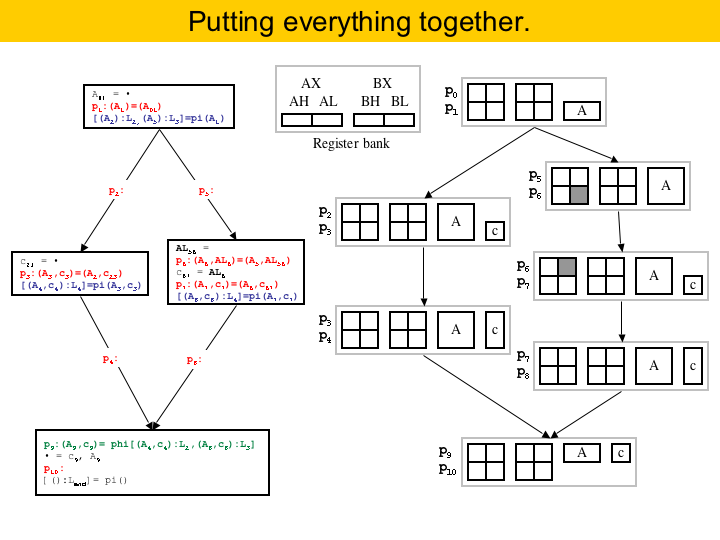To put everything together, on the left we have the program in elementary form, and on the right we have the puzzles produced for this program. In this example architecture we have only two registers, which are divided into pairs. The register bank is illustrated in the upper part of the figure. You may notice that two puzzles have some areas filled already. This is because of pre-assigned registers. Some conventions in the target architecture force some variables to be stored in particular registers. An example is the division instruction in X86. Div requires the dividend to be in register AX. The remainder is stored into AH, and the quotient is stored into AL.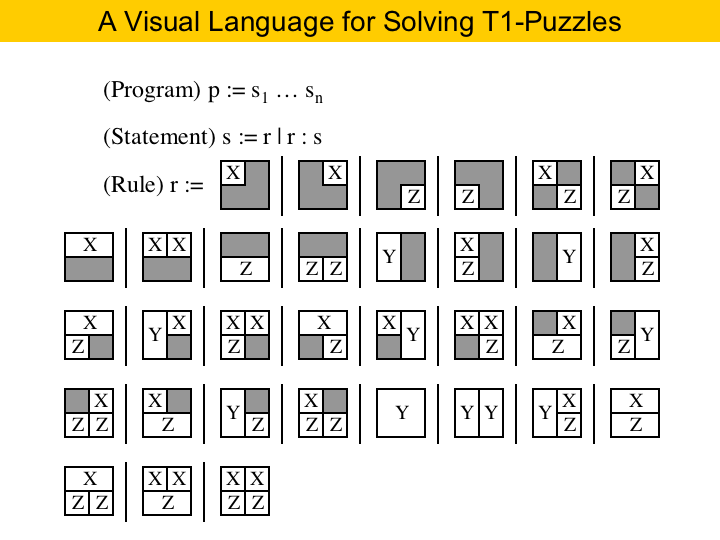Now, we face the problem of solving type-1 puzzles. Well, these are not very complicated puzzles, as each area has only four boxes that must be filled. This means that the number of patterns in which you can fill an area is not too big. In this figure we have listed all the different ways to fill up an area. You may have realized that this figure is indeed a grammar. This is our visual language for programming type-1 puzzle solvers. A program is a sequence of statements, as you can see in the first rule. Each statement is either a rule 'r', or a conditional statement. A rule is a pattern. Patterns are determined by the pre-coloring of the area. The gray boxes mark pre-colored boxes. If you remember, we have pre-coloring because of the use of fixed registers in the source program. Before we move on, let me point something out. Our puzzle solving engine solves one area at a time. It solves an area by filling it up with pieces. For the program to work, the area of the pieces must equal the area of the empty boxes. If the area of the pieces is greater, than we cannot solve the puzzle. If the area of the pieces is smaller, we do some padding. That means that we add some size-1 pieces of type X and Z to the puzzle, until we have the same area.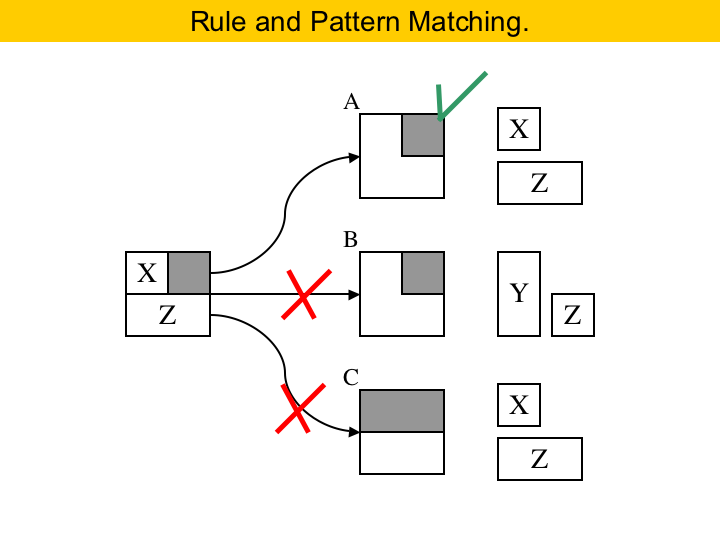A rule explains how to complete an area. It works by pattern matching. For instance, here at the left we have a rule. It says that, if the puzzle contains an area where the upper-right corner is already filled, and the puzzle contains a size-2 Z piece plus a size-1 X piece, than we can complete the area. So, we can apply this rule in the situation A, above in the Figure. But we cannot apply this rule in situation B. You see, the puzzle in B is solvable, but not by this rule, because the pieces are different, although the pattern is the same. Also, we cannot apply this rule in situation C. The problem now is that the patterns do not match.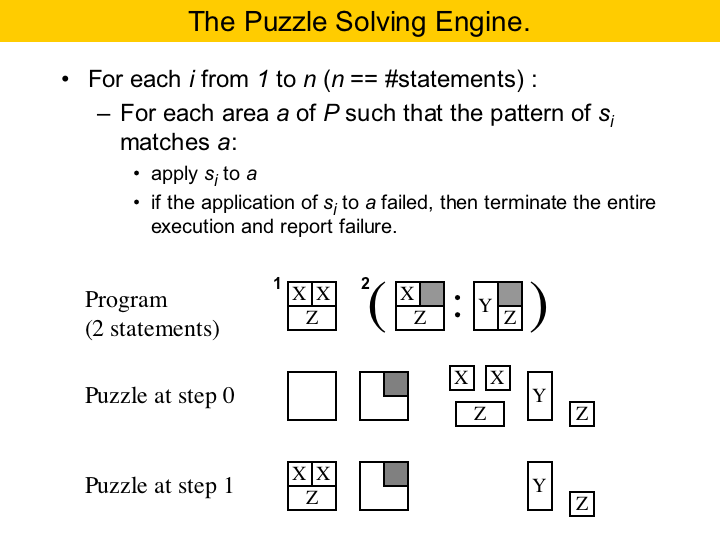So, this figure shows our puzzle solving engine. Again, our algorithm solves one area completely at each time. A puzzle solving program is a collection of statements. In this example here, we have a program with two statements. The second statement is a conditional. It means that, when visiting an area, first we try to apply the first rule, and then the second. All the rules in a conditional statement must have the same pattern. Let's see how this program does on this example puzzle here. The solver iterates twice, once for each statement. First, it tries to apply the rule in statement One. We can do this in the first incomplete area of the puzzle. We have an area where the pattern matches, and we have all the pieces that we need. So, we apply the first rule, we complete the area and remove the pieces from the puzzle. After we visit all the areas, trying to apply the first statement, if there are still pieces adrift, we go for the second statement. The second statement matches the second area in the puzzle. We cannot use the first rule of the second statement, because the pieces do not match. However, we can use the second, and so we do it. There is no more statements to use, but also there is no more free pieces, and we are done. What is the complexity of this solver. Well, we have two loops, first we go over the statements in the solver, and for each statement we have to iterate on the unfilled areas. But notice that the number of possible statements is bounded. This implies that the first iteration will happen a constant number of times per puzzle solving program. Then, the overall complexity is linear on the number of empty areas of the puzzle.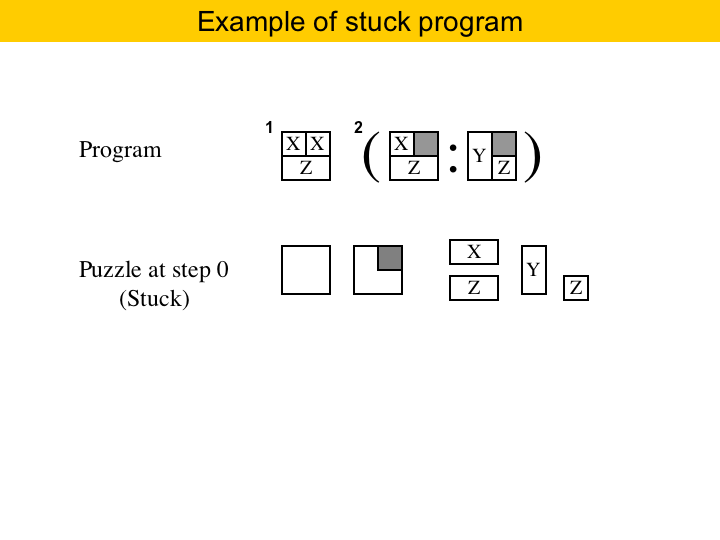Of course, a program may not be able to solve a puzzle. The same program used before would not solve this puzzle here. The pattern in the first statement matches the empty area, but the pieces do not match. So this program is stuck. If a statement gets stuck, then we stop the solver, and report that there is no solution.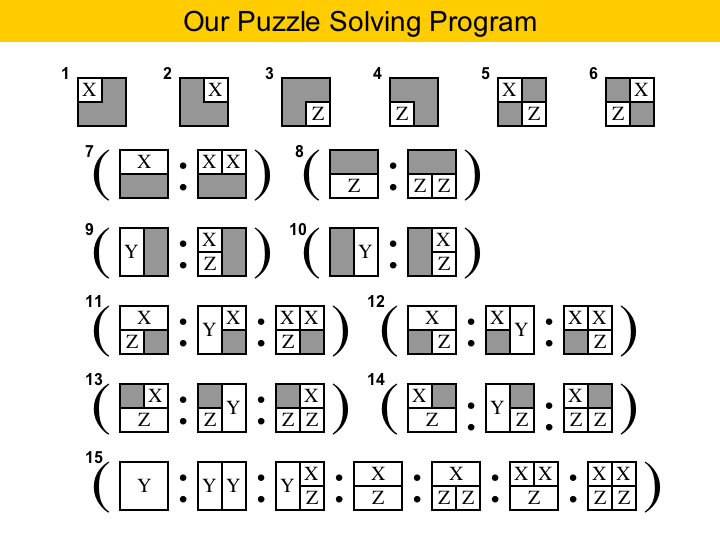And here we have our puzzle solving program. We have fifteen statements, each of which complete an area with a different pattern. Because each area has four boxes, we can have sixteen different patterns, but one of them, the area complete filled, does not have to be taken into consideration. So, what is the rationale for ordering the statements like this? You see, first we have to try to fill all the areas that have already three boxes pre-colored, then two boxes in diagonal, and so on. What is this ordering based upon? In a way, we solve the most constrained areas first. The ordering is indeed very important: if you change it, the program may no longer work, as we will show in some examples.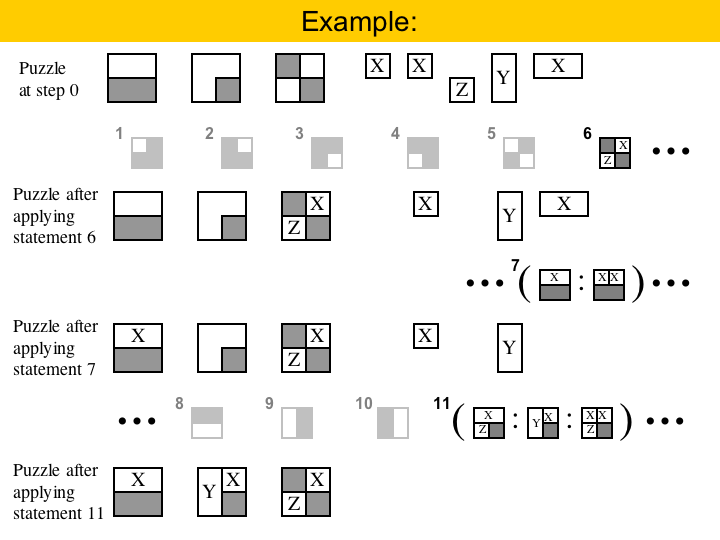Let's see how this program works. On the top here we have a puzzle with three areas, and five pieces. To solve this puzzle, we must go over each statement in my program. The first five statements do not match any of the areas, but the sixth does. We can use statement six to solve the third area, because we have the pieces available. Statement six only applies in one area, and after applying it, we can move on to the next statement. Statement seven matches the first area, and we have the big X piece available, so we can fill that area. Statements 8, 9 and 10 do not produce any match, but statement 11 does. We cannot use the first rule, because we do not have the pieces, but we can use the second. After that, there is no pieces to be placed, and we are done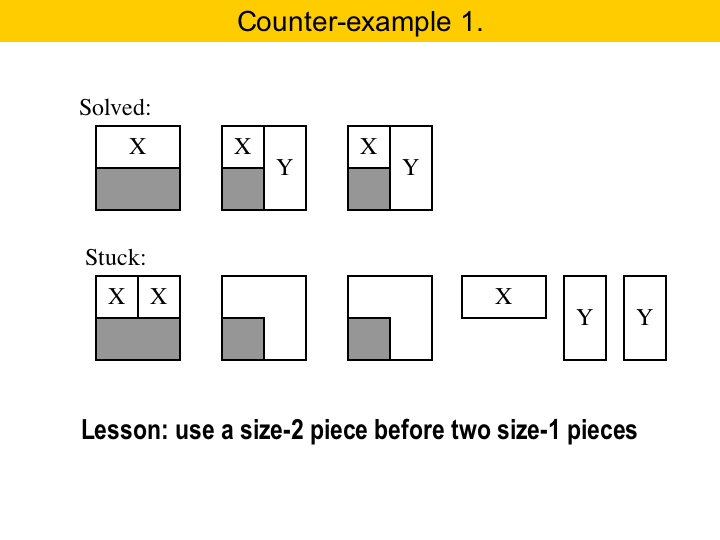The next four slides show some subtleties on the ordering of our puzzle solving program. First, in statement seven you see that we must try to use a size-2 X piece before we try to use two size-1 X pieces. If we did otherwise, we could get stuck, as in this example here.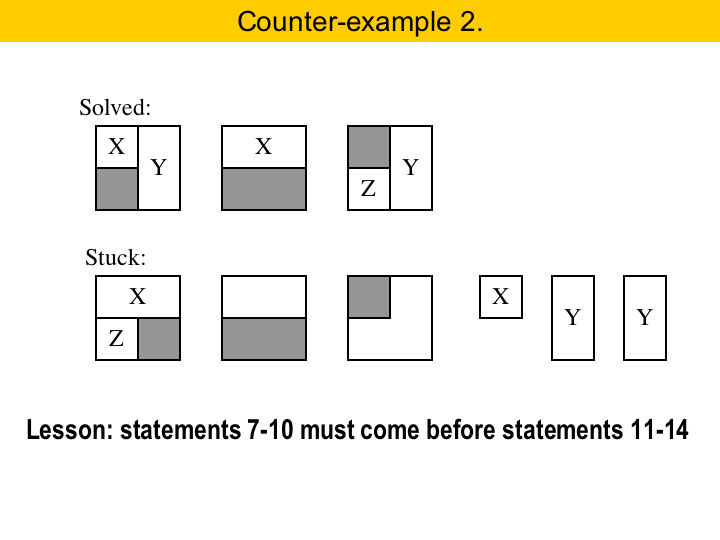When we have a size-2 X piece, it is always better to try to inserted in into an area with the upper row already filled. This is what statement seven says. Because we statements seven to ten come before 11 to 14, this will always happen. However, if we applied statement 11 before statement seven we could get a stuck puzzle.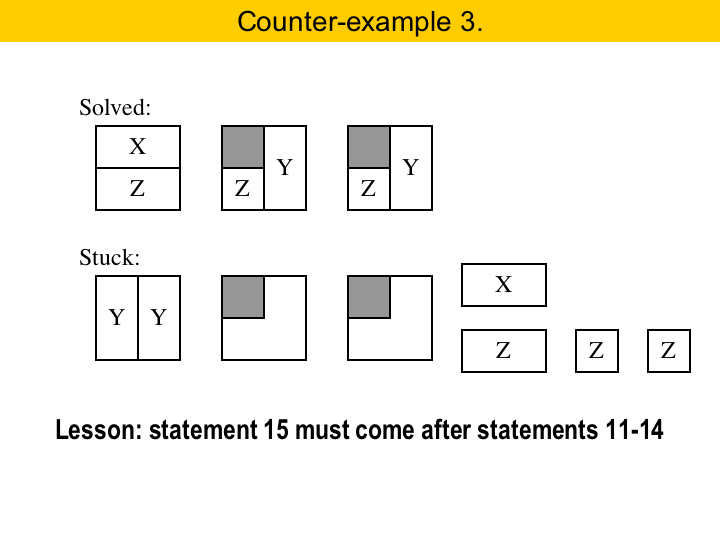The areas with the gray box are more constrained, so they should be solved first. That is way statements 11 to 14 come before statement 15. If that were not the case, we could get unnecessarily stuck, as we show here in the example. In this case, we should save the Y pieces for the areas with the gray boxes. Because we did not do this, we got stuck.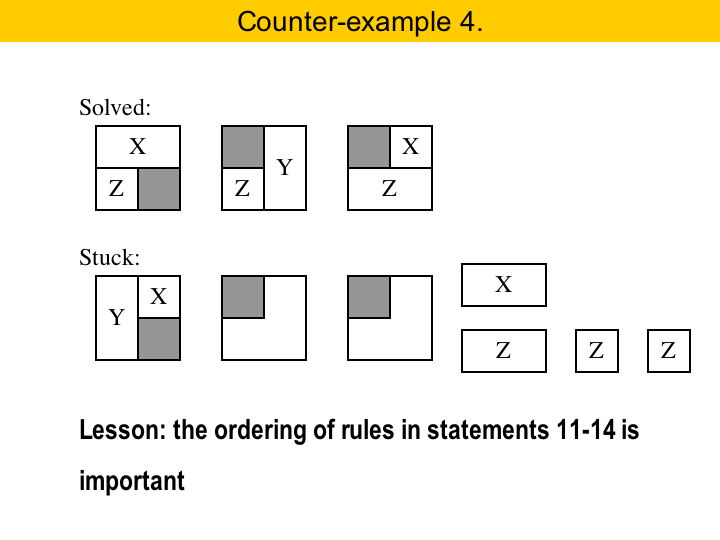Our last counter-example. This one here is quite subtle. Statements 11 to 14 are conditionals. They have three rules each, and these rules really must be applied in the order that they were specified. For instance, here, we solve the first area with a size-2 X piece. If, instead, we had used the size-2 Y piece, we would get stuck. So, even though these seem like symmetric arrangements, they are not. The point is that a size-2 X piece is more constrained: it cannot move inside an area, whereas the size-2 Y piece can. So, we should always try to get rid of size-2 X pieces before eleminating size-2 Y pieces.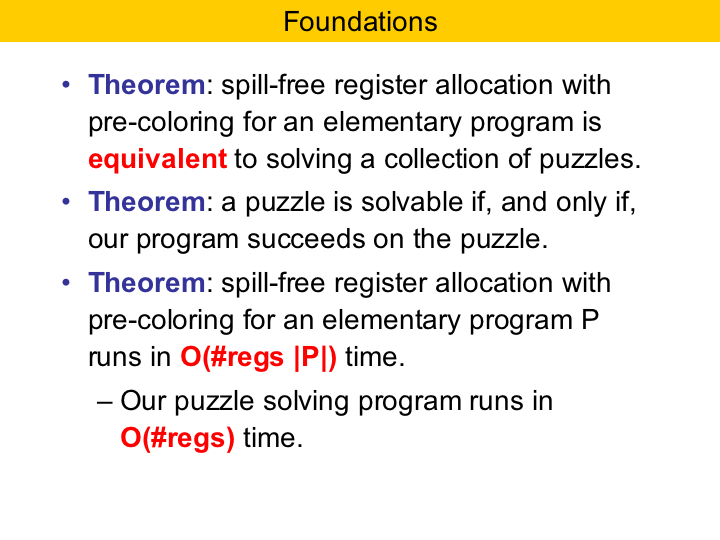We do not elaborate much on the theory behind the puzzles, but this theory certainly exists. Our long paper contains three main theorems dealing with type-1 puzzles, that we prove in the extended version of the paper. The first is an equivalence theorem, where we show that puzzle solving is indeed equivalent to register allocation with aliasing and pre-colored registers. The second theorem states that the program that we use to proof type-1 puzzles is correct. The main technique to proof that this theorem is right is based on swaps of pieces. Basically we assume that the puzzle has a solution, and show how, by swapping pieces we can get from the solution to the solution stated by the puzzle solver. The third theorem combines the first two to show that we can find an optimal register assignment in time proportional to the number of registers times the size of the program.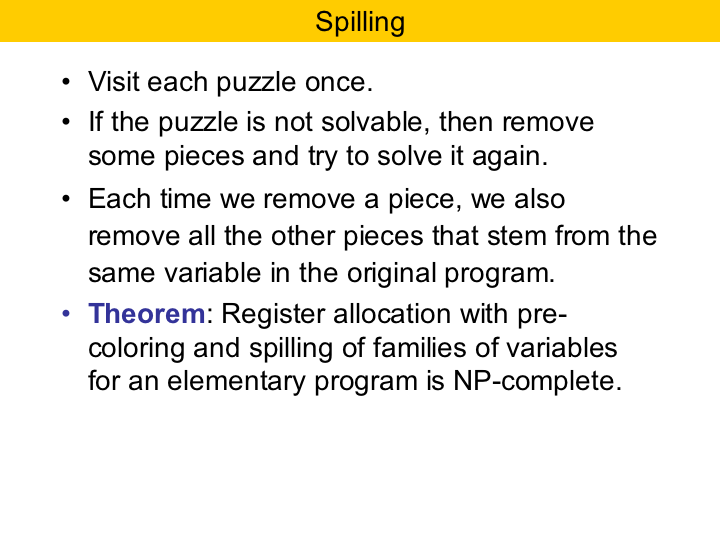Now you may be wondering how spilling plays a role in our abstraction. Sometimes we do not have enough registers, and then some variables must be sent to memory. This is called spilling. In our implementation of the puzzle based register allocator we visit each instruction only once. If we can solve the puzzle of that instruction, than that is it, and we move on to the next instruction. However, if we cannot solve the puzzle, we must choose a piece and send it to memory. Then we must solve the puzzle again. We repeat this process until we get a solvable puzzle. The question now is which piece to remove. Well, first of all, we cannot remove X or Z pieces. These pieces are used in the instruction that underlies the puzzle. So, we must remove a Y piece, and we choose that one that is the farthest to be used. This is called the Belady's heuristics, and it is used in the linear scan register allocator. Once a piece is removed, we do not have to consider that variable in future puzzles, as long as the variable is not used in any instruction. If we have to reload a variable, then we keep it in registers, until we have to spill it again, or we no longer need it. Of course, our approach is a heuristics. Finding which variables to remove in order to minimize the number of loads and stores in the target program is a NP-complete problem, as we state in another theorem in the paper.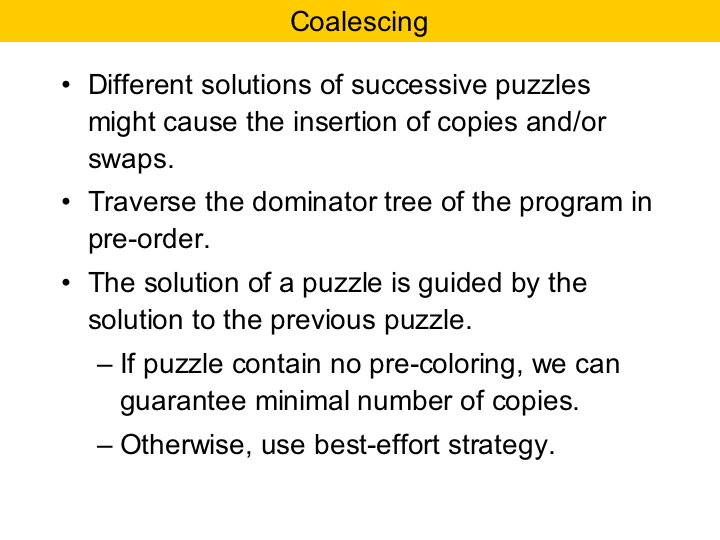Another point that is important in register allocation is coalescing. That is, we must try to allocate pieces that represent the same variable into the same register along successive instructions. That is because if a piece gets assigned to a register in one puzzle, and a different register in the next puzzle, then we will have to insert some copy or swap to keep the program correct. The problem of finding a register assignment that minimizes the number of copies in the whole program is NP-complete. However, from one puzzle to the other we can do much better. If the puzzle contains no pre-coloring, then finding a register assignment that minimizes the number of copies can be done in polynomial time. If we have pre-coloring, then we do not know the complexity class, and we use a best-effort strategy. So, in our implementation we traverse the dominator tree of the source program. The solution of a puzzle is guided by the solution of the previous puzzle. We call this local coalescing. We do not do much for global coalescing, that is, minimizing the number of copies in the whole program, because this problem is NP-complete, and we were implementing a fast register allocator. But there is nothing that prevents a different register allocator of using more advanced strategies and global information to solve the puzzles.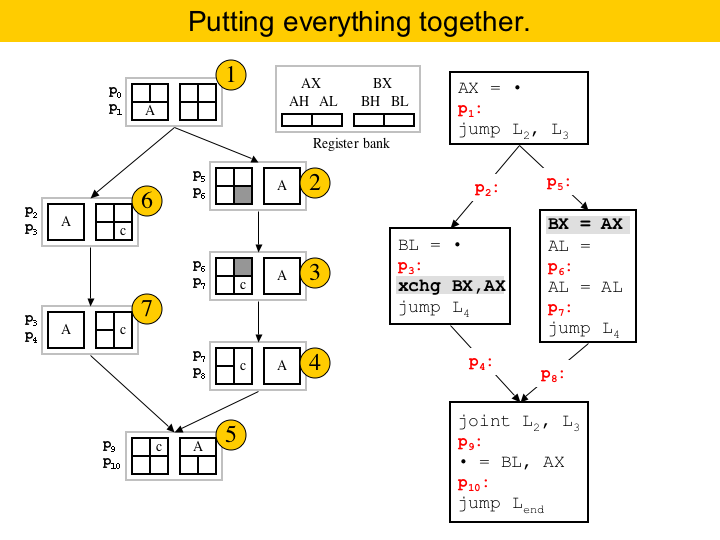So, lets wrap it up by showing how our running example can be solved using puzzles. We have seven puzzles, and we solve them in the order indicated by the numbers in gold. There are two things that we should say here. First, you see that from one puzzle to the other we can do a good job by assigning registers to pieces in such a way to avoid inserting copies between puzzles. The second is that, because we do not use global information, we had to insert two new instructions in the program. This copy in the right basic block, and the swap at the end of the left basic block. If we were using global information, like the interference graph of variables, we would avoid assigning variable A to register AX, because of the precolored area in puzzles 2 and 3. But in this case we would have to build the interference graph, and the algorithm would be a slower.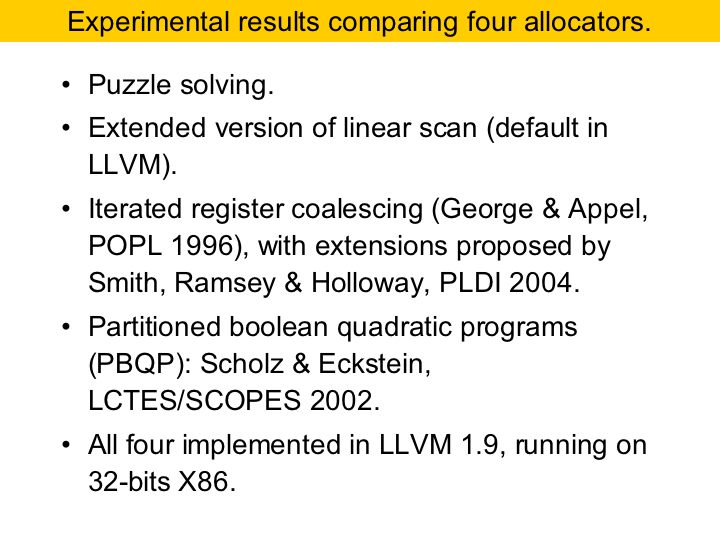Now, lets talk about some experiments. We compared four different register allocators, all of them implemented in LLVM, version 1.9. LLVM is a very nice compiler framework. It is used, for instance, as a JIT compiler in Mac OS 1.5. Besides the puzzle solver, the other algorithms that we have used were an extended version of linear scan, a graph coloring algorithm and an exponential time algorithm called PBQP. The extended version of linear scan is used by LLVM as the default register allocator. It fast, and produces good code. It fills roles in the live ranges of variables with other variables, and it backtracks if spills happen. The other algorithms were implemented by our friends from the university of Sidney: Lang Hames and Bernhard Scholtz.We have done a lot of testing. LLVM provides a benchmark suite that, together with SPEC CPU 2000 gave us about 1.3 million lines of C code. We will be showing the results for SPEC 2000. This is about 600K lines of C code. LLVM does some inlining, and the assembly code in general tends to be quite big..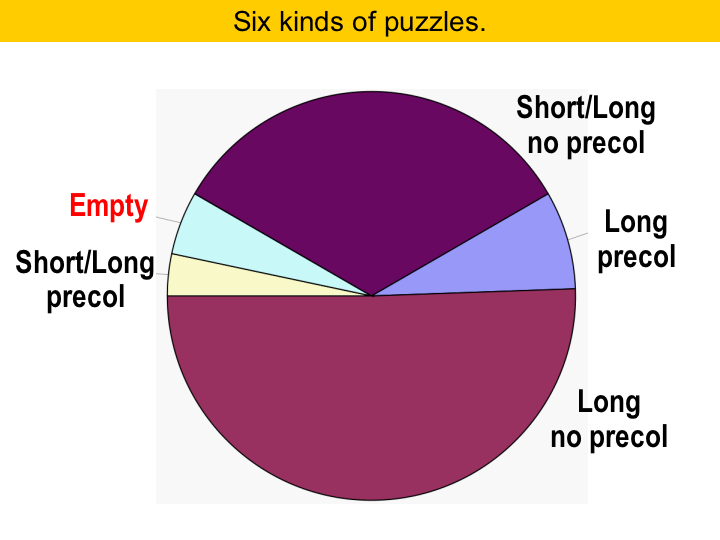This table shows which types of puzzles we had to deal with. We call 16 or 32-bit values long, and 8-bit values short. Remember that the long values produce the pieces spanning two columns. You may notice that SPEC does not use 8-bit values that often. A little more than half the puzzles had only long values. Also, there were many empty puzzles, that is, puzzles which have a board and no pieces. The board may contain some pre-coloring. About one third of the puzzles had shorts and longs.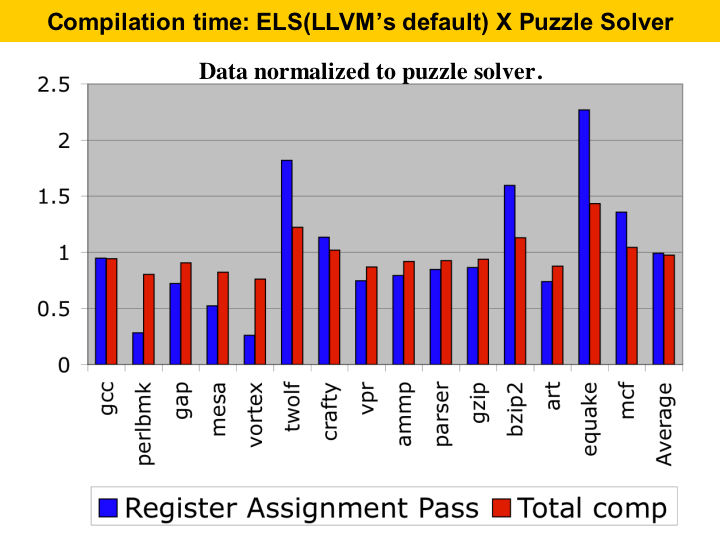This graph here shows compilation time. We are comparing the puzzle solver with the extended version of linear scan used by LLVM. The bars show the time of linear scan, and they are normalized to the puzzle solver. The blue bar gives the time of the register assignment phase only, while the red bar gives the full compilation time. On the average, both algorithms have very similar compilation time. The puzzle solver is less than 2% slower than LLVM�s default algorithm. When considering total compilation time, the difference is about 3%. The increase is because of the extra-phase to remove critical edges and to eliminate phi-functions.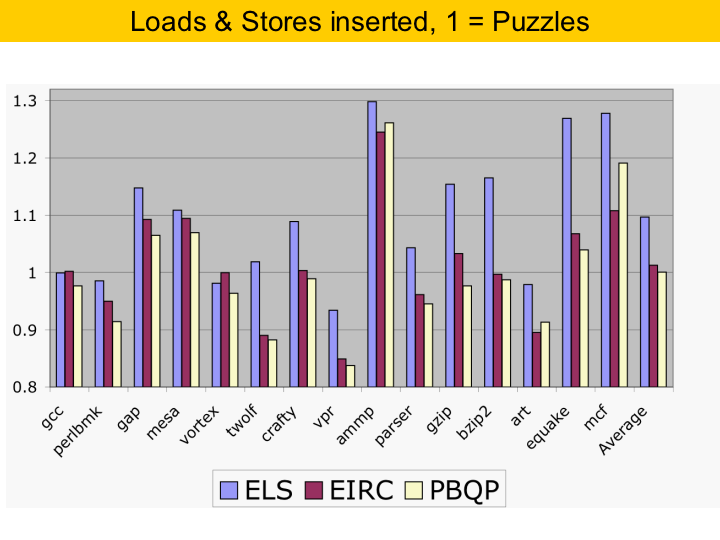Here we compare the static number of loads and stores that each algorithm inserted into the code. The bars are normalized to the puzzle solver. The code produced by the puzzle solver had fewer memory accesses than the other algorithms, although the difference with PBQP was very small.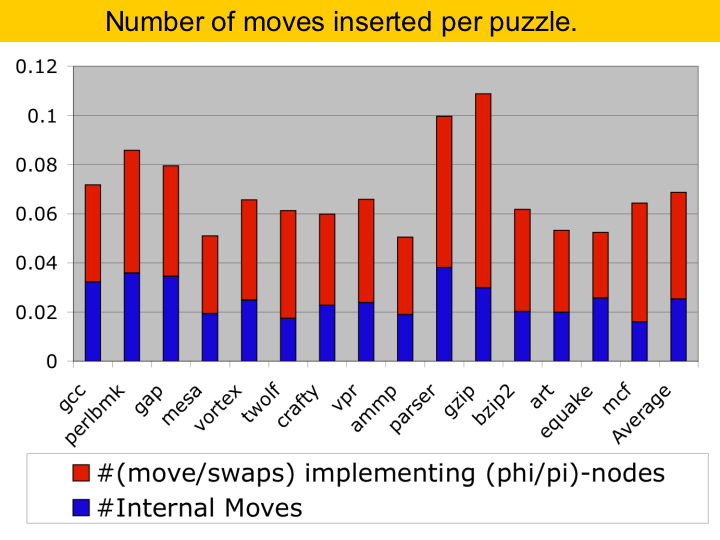This chart here shows the number of move instructions inserted by the puzzle solver. On the average we had to insert one move per about each 14 instructions. The blue part of the bars shows the moves inserted between consecutive puzzles inside the same basic bloc, and the red part shows the moves and swaps inserted during SSA-elimination, when we had to replace phi-functions with move instructions.This is our last chart. It compares the execution time of the the code produced by each algorithm. One thing to notice is that the difference between the algorithms is very small. This is because the algorithms are already very good, and it is difficult to improve much on performance, given how good they were. Yet, the puzzle solver is only slower than PBQP, that takes much longer compilation time. These bars are normalized with GCC -O3. One thing that we realized is that the puzzle solver tends to produce faster code in programs that have sparce control flow graph. For programs with big basic blocks it is very, very good. For programs with switch statements it does not do so well.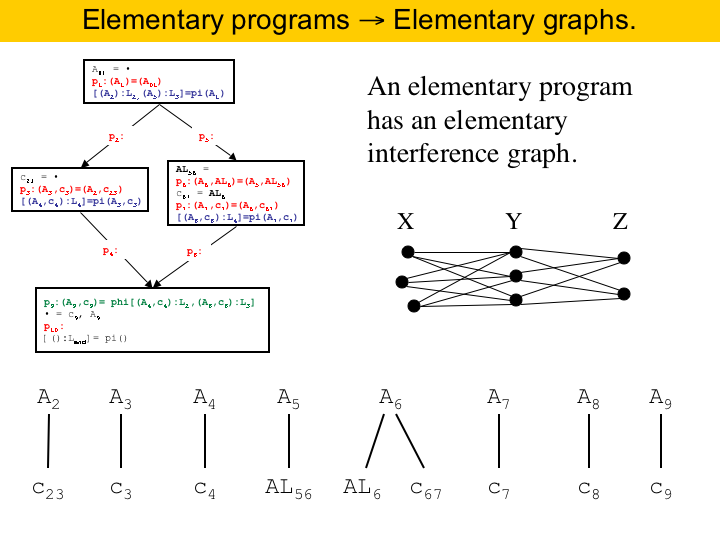Now, before closing, lets just give a glimpse on the theory of register allocation by puzzle solving. The interference graph of an elementary program is called an elementary graphs. Elementary graphs are very simple. Each connected component of an elementary graph is a clique substitution of P3. There is an example here at the right. The interference graph for our running program is given below here.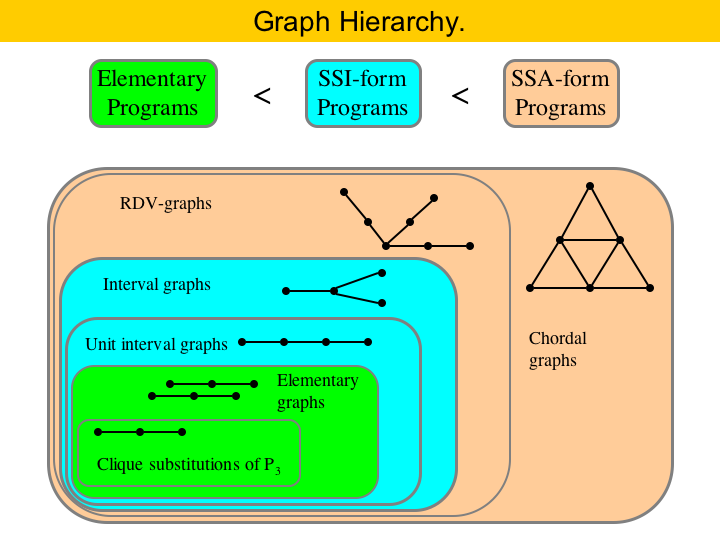Different representations of programs produce different interference graphs. General programs have general interference graphs. That is, since the early eighties we know that any graph is the interference graph of some general program. If we impose some restrictions on our representation, we can get smaller classes of graphs. For instance, SSA-form programs have chordal interference graphs. This is known since 2005. If the program is in SSI form, than its interference graph is an interval graph. Interval graphs are a subfamily of the chordal graphs. Finally, if the program is in elementary form, its interference graph is called an elementary graph. Elementary graphs are a subfamily of the interval graphs. This hierarchy is shown in the Figure here.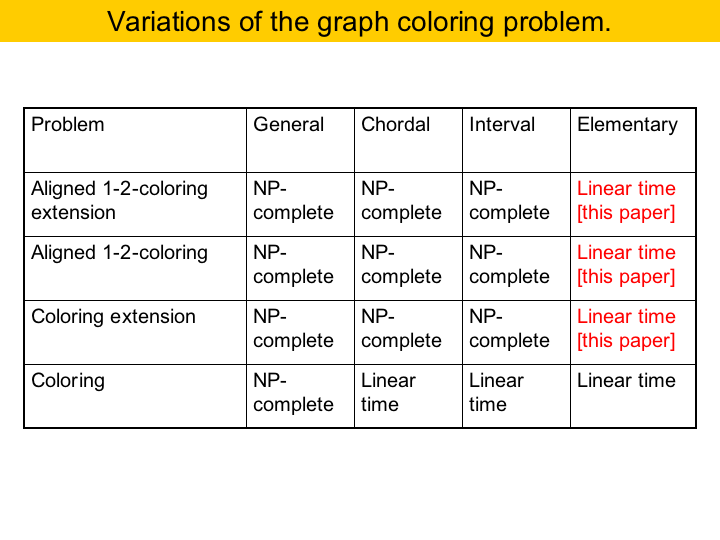Register assignment can be modeled by different instances of the graph coloring problem. The general register assignment problem, that is, given a program, what is the minimal number of registers necessary to compile it, has polynomial solution for SSA-form programs, because coloring chordal graphs is solvable in polynomial time. If I have pre-colored registers, then the the closer problem is called pre-coloring extension. I have a graph, with some nodes pre-colored, and I want to extend this coloring to the full graph. This problem is NP-complete for chordal and interval graphs, but it has polynomial time solution for elementary graphs. Also, if I have register pairs, the equivalent problem is coloring of weighted graphs with weights of 1 and 2. This is polynomial for elementary graphs, but is NP-complete for the other classes. The combination of these two problems, pre-coloring extension and weighted coloring is polynomial for elementary graphs, and the algorithm is the puzzle solving approach that we gave in this presentation.So, that is register allocation by puzzle solving. We believe that it is a simple and elegant approach to register allocation. One important thing is that, if it is possible to find a register assignment that does not cause any spilling, then the puzzle solver will find it. Register allocation by puzzle solving is as fast as modern versions of linear scan, and it produces code as good as the code produced by graph coloring algorithms.Última atualização: 11 de Junho de 2008.

Last update: June 11th, 2008.